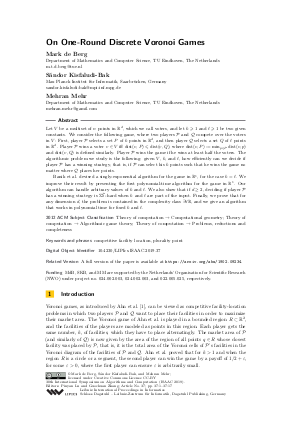Document# On One-Round Discrete Voronoi Games

### Authors Mark de Berg, Sándor Kisfaludi-Bak, Mehran Mehr## File

LIPIcs.ISAAC.2019.37.pdf
• Filesize: 0.69 MB
• 17 pages

## Cite As

Mark de Berg, Sándor Kisfaludi-Bak, and Mehran Mehr. On One-Round Discrete Voronoi Games. In 30th International Symposium on Algorithms and Computation (ISAAC 2019). Leibniz International Proceedings in Informatics (LIPIcs), Volume 149, pp. 37:1-37:17, Schloss Dagstuhl - Leibniz-Zentrum für Informatik (2019)
https://doi.org/10.4230/LIPIcs.ISAAC.2019.37

## Abstract

Let V be a multiset of n points in R^d, which we call voters, and let k >=slant 1 and l >=slant 1 be two given constants. We consider the following game, where two players P and Q compete over the voters in V: First, player P selects a set P of k points in R^d, and then player Q selects a set Q of l points in R^d. Player P wins a voter v in V iff dist(v,P) <=slant dist(v,Q), where dist(v,P) := min_{p in P} dist(v,p) and dist(v,Q) is defined similarly. Player P wins the game if he wins at least half the voters. The algorithmic problem we study is the following: given V, k, and l, how efficiently can we decide if player P has a winning strategy, that is, if P can select his k points such that he wins the game no matter where Q places her points. Banik et al. devised a singly-exponential algorithm for the game in R^1, for the case k=l. We improve their result by presenting the first polynomial-time algorithm for the game in R^1. Our algorithm can handle arbitrary values of k and l. We also show that if d >= 2, deciding if player P has a winning strategy is Sigma_2^P-hard when k and l are part of the input. Finally, we prove that for any dimension d, the problem is contained in the complexity class exists for all R, and we give an algorithm that works in polynomial time for fixed k and l.

## Subject Classification

##### ACM Subject Classification
• Theory of computation → Computational geometry
• Theory of computation → Algorithmic game theory
• Theory of computation → Problems, reductions and completeness
##### Keywords
• competitive facility location
• plurality point

## Metrics

• Access Statistics
• Total Accesses (updated on a weekly basis)
0

## References

1. Hee-Kap Ahn, Siu-Wing Cheng, Otfried Cheong, Mordecai Golin, and Rene Van Oostrum. Competitive facility location: the Voronoi game. Theoretical Computer Science, 310(1-3):457-467, 2004.2. Miklós Ajtai, János Komlós, and Endre Szemerédi. Sorting in c log n parallel steps. Combinatorica, 3(1):1-19, 1983.3. Aritra Banik, Bhaswar B Bhattacharya, and Sandip Das. Optimal strategies for the one-round discrete Voronoi game on a line. Journal of Combinatorial Optimization, 26(4):655-669, 2013.4. Aritra Banik, Bhaswar B Bhattacharya, Sandip Das, and Sreeja Das. Two-round discrete Voronoi Game along a line. In Frontiers in Algorithmics and Algorithmic Aspects in Information and Management, pages 210-220. Springer, 2013.5. Aritra Banik, Bhaswar B Bhattacharya, Sandip Das, and Satyaki Mukherjee. The discrete Voronoi game in R2. Computational Geometry, 63:53-62, 2017.6. Aritra Banik, Jean-Lou De Carufel, Anil Maheshwari, and Michiel Smid. Discrete Voronoi games and epsilon-nets, in two and three dimensions. Computational Geometry, 55:41-58, 2016.7. Saugata Basu, Richard Pollack, and Marie-Françoise Roy. On the combinatorial and algebraic complexity of quantifier elimination. Journal of the ACM, 43(6):1002-1045, 1996.8. Otfried Cheong, Sariel Har-Peled, Nathan Linial, and Jiří Matoušek. The One-Round Voronoi Game. Discrete & Computational Geometry, 31(1):125-138, 2004.9. Mark de Berg, Joachim Gudmundsson, and Mehran Mehr. Faster algorithms for computing plurality points. ACM Transactions on Algorithms, 14(3):36:1-36:23, 2018.10. Michael G Dobbins, Linda Kleist, Tillmann Miltzow, and Paweł Rzążewski. ∀ ∃ R-Completeness and Area-Universality. In Proceedings of the 44th International Graph-Theoretic Concepts in Computer Science (WG 2018), pages 164-175, 2018.11. Sándor P Fekete and Henk Meijer. The one-round Voronoi game replayed. Computational Geometry, 30(2):81-94, 2005.12. David Lichtenstein. Planar formulas and their uses. SIAM Journal on Computing, 11(2):329-343, 1982.13. Wei-Yin Lin, Yen-Wei Wu, Hung-Lung Wang, and Kun-Mao Chao. Forming Plurality at Minimum Cost. In Proceedings of the 9th International Workshop on Algorithms and Computation, pages 77-88, 2015.14. Richard D McKelvey and Richard E Wendell. Voting equilibria in multidimensional choice spaces. Mathematics of operations research, 1(2):144-158, 1976.15. Michael Paterson and Uri Zwick. Shallow circuits and concise formulae for multiple addition and multiplication. Computational Complexity, 3(3):262-291, 1993.16. Joachim Spoerhase and H-C Wirth. (r, p)-centroid problems on paths and trees. Theoretical Computer Science, 410(47-49):5128-5137, 2009.17. Larry J Stockmeyer. The polynomial-time hierarchy. Theoretical Computer Science, 3(1):1-22, 1976.18. Sachio Teramoto, Erik D Demaine, and Ryuhei Uehara. The Voronoi game on graphs and its complexity. Journal of Graph Algorithms and Applications, 15(4):485-501, 2011.19. Yen-Wei Wu, Wei-Yin Lin, Hung-Lung Wang, and Kun-Mao Chao. Computing plurality points and Condorcet points in Euclidean space. In International Symposium on Algorithms and Computation, pages 688-698, 2013.X

Feedback for Dagstuhl Publishing